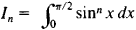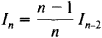# Recurrence Formula

## Recurrence Formula

(or recursion formula), a formula that reduces the computation of the nth term of some sequence (most often, a numerical sequence) to the computation of one or more of the terms preceding the nth term. The relevant preceding terms are usually found “near” the nth term, the number of the relevant preceding terms is independent of n, and the nth term can be expressed by means of these terms in a relatively simple manner. Recurrence formulas of a more complex nature, however, are possible. The general problems involved in recursion computations are the subject of the theory of recursive functions.

Examples. (1) The sequence Φn of Fibonacci numbers is defined by the formulas

Φ0 = 0

Φ1 = 1

Φπ+2 = Φπ+1 + Φπn ≥ 0

The last of these formulas is a recurrence formula and can be used to compute Φ2, Φ3, and subsequent terms of the sequence.

(2) SupposeIt can be easily proved that if n ≥ 2,This recurrence formula reduces the calculation of In to the calculation of I0 or I1 depending on whether η is odd or even.

Recurrence formulas usually provide a convenient computational scheme for finding the terms of a sequence one after another. Sometimes, however, an attempt is made to obtain on the basis of recurrence formula an “explicit” expression for the nth term of the sequence described by the formula. Thus, in the case of the Fibonacci numbers,References in periodicals archive ?
And a practical method is proposed to determine the minimum embedding dimension and get the recurrence formula, with which a capacity model is built.
In particular, we obtain a very efficient recurrence formula that can be used to compute the generating function of maps of fixed genus inductively.
Once the students discover the recurrence formula for the series coefficients, it's requested to build a Classpad300 program to automatically generate terms of the series.
We shall give a bijective proof and an analytical proof of (9), the latter leads to a new recurrence formula for [A.
The Bl-BCG algorithm uses a short three-term recurrence formula, but in many situations the algorithm exhibits a very irregular convergence behavior.
The following recurrence formula for FA(q) can be found in Borodin (1995), where he considers the matrices as particles of a certain mass.
We also express the recurrence formula for [MATHEMATICAL EXPRESSION NOT REPRODUCIBLE IN ASCII] in terms of Vandermonde type determinants.
Abstract In this paper discussed recurrence formula and sum formulas for the generalised Euler numbers [E.
It is also closely related to the approach that Khamis  has used to derive a recurrence formula for the number of interval orders of a given size and height.
Keywords The Euler numbers, the generalized Euler numbers, recurrence formula.
Keywords Euler numbers, generalized Euler numbers, Bernoulli numbers, recurrence formula.

Site: Follow: Share:
Open / Close